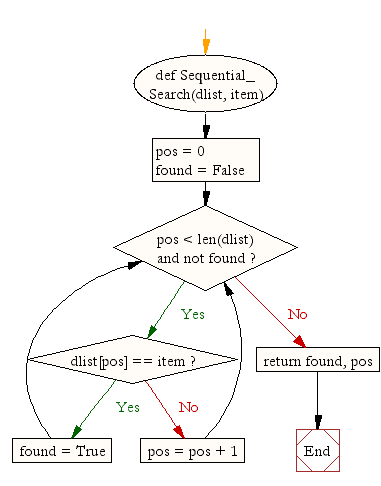﻿ Python Data Structures and Algorithms: Sequential search - w3resource# Python Data Structures and Algorithms: Sequential search

## Python Search and Sorting: Exercise-2 with Solution

Write a Python program for sequential search.
Sequential Search: In computer science, linear search or sequential search is a method for finding a particular value in a list that checks each element in sequence until the desired element is found or the list is exhausted. The list need not be ordered.

Sample Solution:

Python Code:

``````def Sequential_Search(dlist, item):

pos = 0
found = False

if dlist[pos] == item:
found = True
else:
pos = pos + 1

return found, pos

print(Sequential_Search([11,23,58,31,56,77,43,12,65,19],31))
```
```

Sample Output:

```(True, 3)
```

Flowchart:## Visualize Python code execution:

The following tool visualize what the computer is doing step-by-step as it executes the said program:

Python Code Editor :

What is the difficulty level of this exercise?

Test your Python skills with w3resource's quiz

﻿

## Python: Tips of the Day

Returns the maximum value of a list, after mapping each element to a value using the provided function

Example:

```def tips_max(lst, fn):
return max(map(fn, lst))

print(tips_max([{ 'n': 4 }, { 'n': 2 }, { 'n': 8 }, { 'n': 6 }], lambda v : v['n']))
```

Output:

```8
```